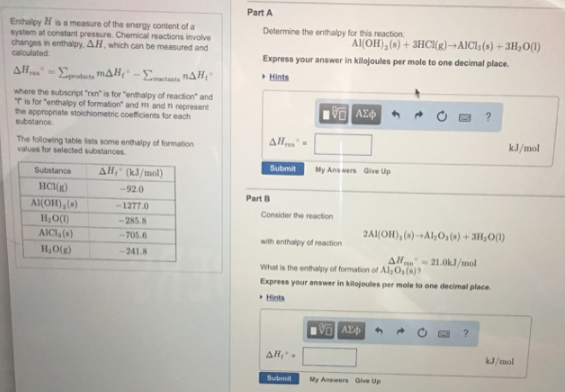# Problem: Enthalpy H is a measure of the energy content of a system at constant pressure. Chemical reactions involve changes in enthalpy, ΔH, which can be measured and calculated: ΔH°rxn = Ʃproducts mΔH°f - Ʃreactants nΔH°f where the subscript "rxn" is for "enthalpy of reaction" and "f" is for "enthalpy of formation" and m and n represent the appropriate stoichiometric coefficients for each substance. The following table lists some enthalpy of formation values for selected substances. Determine the enthalpy for this reaction: Al(OH)3 (s) + 3HCl (g) → AlCl3 (s) + 3H2O (l) Express your answer in kilojoules per mole to one decimal place. Consider the reaction 2Al(OH)3 (s) → Al2O3 (s) + 3H2O (l) with enthalpy of reaction ΔH°rxn = 21.0 kJ/mol What is the enthalpy of formation of Al 2O3(s)? Express your answer in kilojoules per mole to one decimal place.

###### FREE Expert Solution
97% (52 ratings)###### Problem Details

Enthalpy H is a measure of the energy content of a system at constant pressure. Chemical reactions involve changes in enthalpy, ΔH, which can be measured and calculated:

ΔH°rxn = Ʃproducts mΔH°f - Ʃreactants nΔH°f

where the subscript "rxn" is for "enthalpy of reaction" and "f" is for "enthalpy of formation" and m and n represent the appropriate stoichiometric coefficients for each substance.

The following table lists some enthalpy of formation values for selected substances.

Determine the enthalpy for this reaction:

Al(OH)(s) + 3HCl (g) → AlCl(s) + 3H2O (l)

Consider the reaction

2Al(OH)3 (s) → Al2O3 (s) + 3H2O (l)

with enthalpy of reaction

ΔH°rxn = 21.0 kJ/mol

What is the enthalpy of formation of Al 2O3(s)?